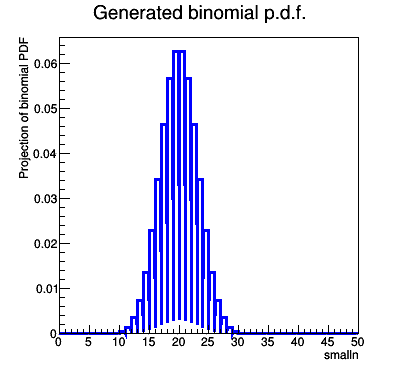# Using RooRealVar in Integer steps

Dear Rooters,

I’m trying to generate a binomial pdf which I can then fit to my measured data. Problem is RooFit doesn’t seem to be able to compute the pdf in discrete integer steps, or to put it another way it has no functionality to allow the user to declare an integer variable, with RooRealVar() implicitly computing the pdf in arbitrary non-integer steps.

Using RooRealVar("","",RooConst(x),RooConst(x1),RooConst(x2)) gives error message …"candidate constructor not viable…

Using RooConstVar() gives similar message.

Is there a workaround? I posted a similar question on the “Stat and Math Tool Support” a few days ago but have had no takers! perhaps I might have better joy here!

see example of code and output below

``````#ifndef __CINT__
#include "RooGlobalFunc.h"
#endif
#include "RooRealVar.h"
#include "RooDataSet.h"
#include "TMath.h"
#include "TCanvas.h"
#include "RooPlot.h"
#include "TAxis.h"
using namespace RooFit ;

void FitGeneratedBinomialModelToData()
{
// Declare variables
RooRealVar p("p","p",0.5,0,1) ;
RooRealVar smalln("smalln","smalln",1,0,50) ;
RooRealVar bigN("bigN","bigN",40,39,41) ;
// build pdf
RooGenericPdf binomialpdf("binomial","binomial PDF","pow(p,smalln)*pow((1-p),(bigN-smalln))*TMath::Factorial(bigN)/(TMath::Factorial(smalln)*TMath::Factorial(bigN-smalln))",RooArgSet(smalln,p,bigN)) ;

// Construct plot frame in 'm'
RooPlot* xframe = smalln.frame(Title("Generated binomial p.d.f.")) ;
// Plot
binomialpdf.plotOn(xframe) ;

// Draw all frames on a canvas
TCanvas* c = new TCanvas("binomialPdf","binomialPdf",400,400) ;
gPad->SetLeftMargin(0.15) ; xframe->GetYaxis()->SetTitleOffset(1.6) ; xframe->Draw() ;
}

******************************
Output:
[attachment=0]binomialPdf.png[/attachment]``````Hi,

You should maybe try to use a binned pdf, such as the RooHistPdf, in this way the integers are taken into accounts as bins

Lorenzo

Hi Lorenzo,

Many thanks for your suggestion. It led to the solution. I generated the binomial function using the TH1F constructor, binned it, imported it into RooDataHist() and then passed it on to RooHistPdf() to give me my pdf. It’s a bit fiddly but it does the trick. The key step is to map the function into the TH1F bins.

For those interested see sample code and output below: